Скачать презентацию Should prices be always lower on the Internet

• Количество слайдов: 8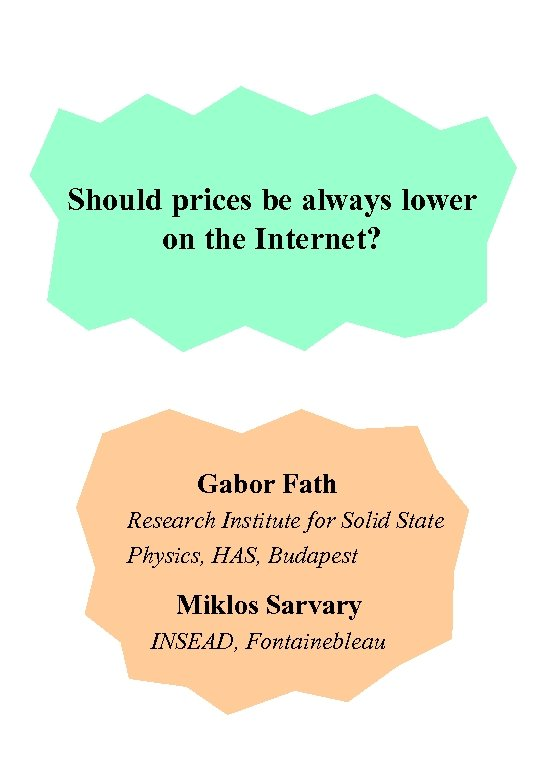Should prices be always lower on the Internet? Gabor Fath Research Institute for Solid State Physics, HAS, Budapest Miklos Sarvary INSEAD, Fontainebleau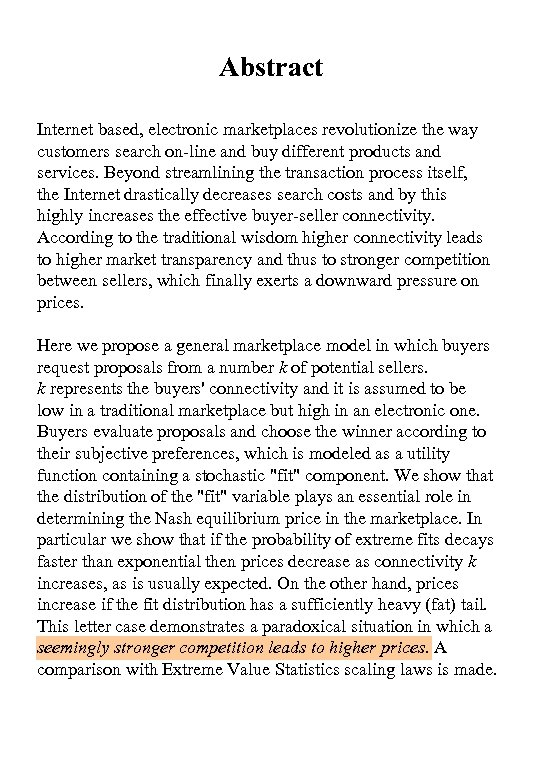Abstract Internet based, electronic marketplaces revolutionize the way customers search on-line and buy different products and services. Beyond streamlining the transaction process itself, the Internet drastically decreases search costs and by this highly increases the effective buyer-seller connectivity. According to the traditional wisdom higher connectivity leads to higher market transparency and thus to stronger competition between sellers, which finally exerts a downward pressure on prices. Here we propose a general marketplace model in which buyers request proposals from a number k of potential sellers. k represents the buyers' connectivity and it is assumed to be low in a traditional marketplace but high in an electronic one. Buyers evaluate proposals and choose the winner according to their subjective preferences, which is modeled as a utility function containing a stochastic "fit" component. We show that the distribution of the "fit" variable plays an essential role in determining the Nash equilibrium price in the marketplace. In particular we show that if the probability of extreme fits decays faster than exponential then prices decrease as connectivity k increases, as is usually expected. On the other hand, prices increase if the fit distribution has a sufficiently heavy (fat) tail. This letter case demonstrates a paradoxical situation in which a seemingly stronger competition leads to higher prices. A comparison with Extreme Value Statistics scaling laws is made.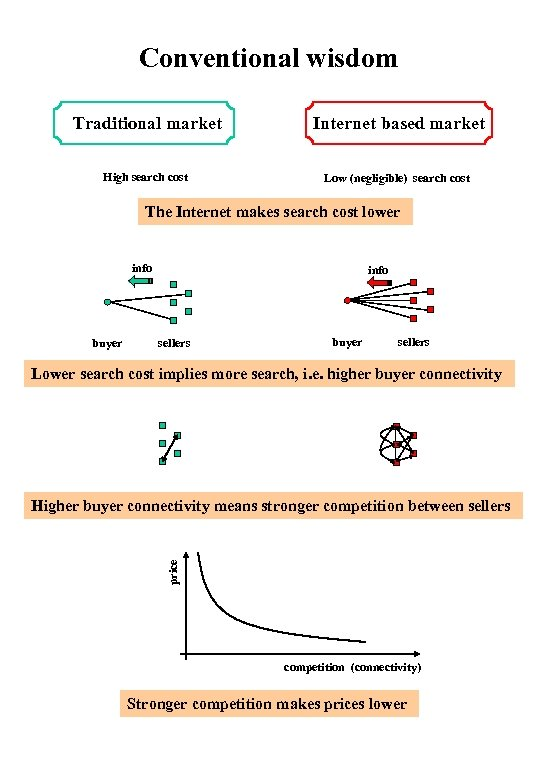Conventional wisdom Traditional market Internet based market High search cost Low (negligible) search cost The Internet makes search cost lower info buyer info sellers buyer sellers Lower search cost implies more search, i. e. higher buyer connectivity price Higher buyer connectivity means stronger competition between sellers competition (connectivity) Stronger competition makes prices lower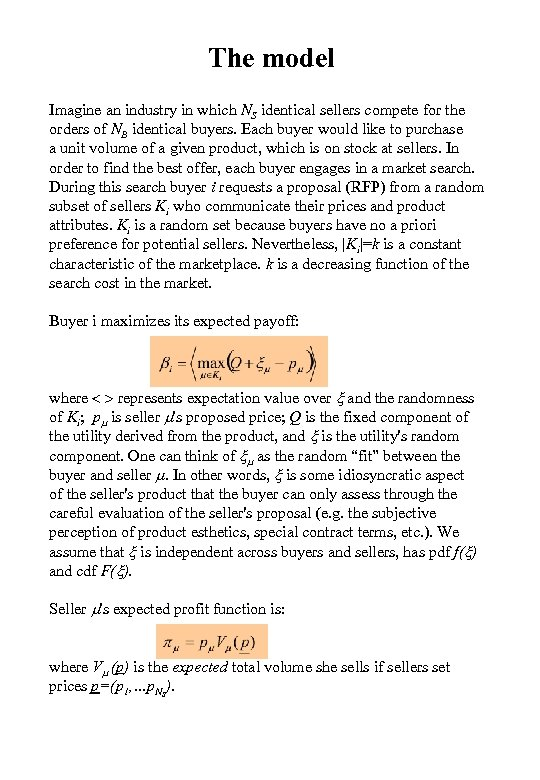The model Imagine an industry in which NS identical sellers compete for the orders of NB identical buyers. Each buyer would like to purchase a unit volume of a given product, which is on stock at sellers. In order to find the best offer, each buyer engages in a market search. During this search buyer i requests a proposal (RFP) from a random subset of sellers Ki who communicate their prices and product attributes. Ki is a random set because buyers have no a priori preference for potential sellers. Nevertheless, |Ki|=k is a constant characteristic of the marketplace. k is a decreasing function of the search cost in the market. Buyer i maximizes its expected payoff: where < > represents expectation value over x and the randomness of Ki; pm is seller m's proposed price; Q is the fixed component of the utility derived from the product, and x is the utility's random component. One can think of xm as the random “fit” between the buyer and seller m. In other words, x is some idiosyncratic aspect of the seller's product that the buyer can only assess through the careful evaluation of the seller's proposal (e. g. the subjective perception of product esthetics, special contract terms, etc. ). We assume that x is independent across buyers and sellers, has pdf f(x) and cdf F(x). Seller m's expected profit function is: where Vm (p) is the expected total volume she sells if sellers set prices p=(p 1, …p. NS).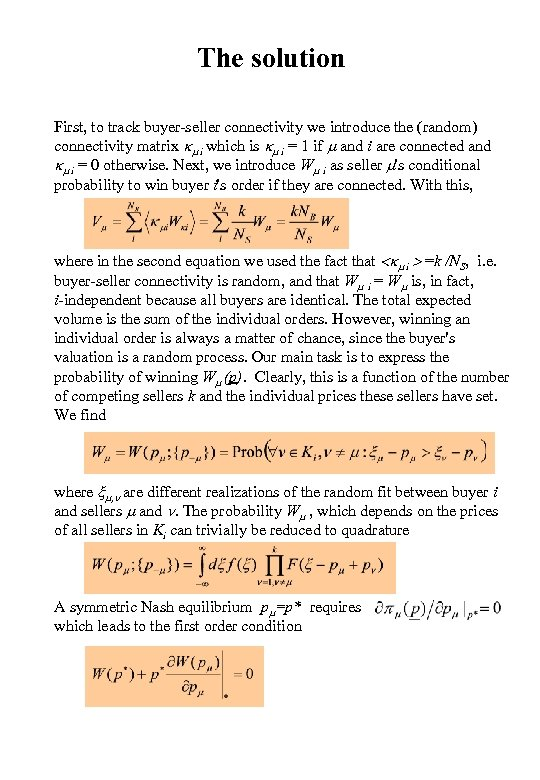The solution First, to track buyer-seller connectivity we introduce the (random) connectivity matrix km i which is km i = 1 if m and i are connected and km i = 0 otherwise. Next, we introduce Wm i as seller m's conditional probability to win buyer i's order if they are connected. With this, where in the second equation we used the fact that =k /NS, i. e. buyer-seller connectivity is random, and that Wm i = Wm is, in fact, i-independent because all buyers are identical. The total expected volume is the sum of the individual orders. However, winning an individual order is always a matter of chance, since the buyer's valuation is a random process. Our main task is to express the probability of winning Wm (p). Clearly, this is a function of the number of competing sellers k and the individual prices these sellers have set. We find where xm, n are different realizations of the random fit between buyer i and sellers m and n. The probability Wm , which depends on the prices of all sellers in Ki can trivially be reduced to quadrature A symmetric Nash equilibrium pm=p* requires which leads to the first order condition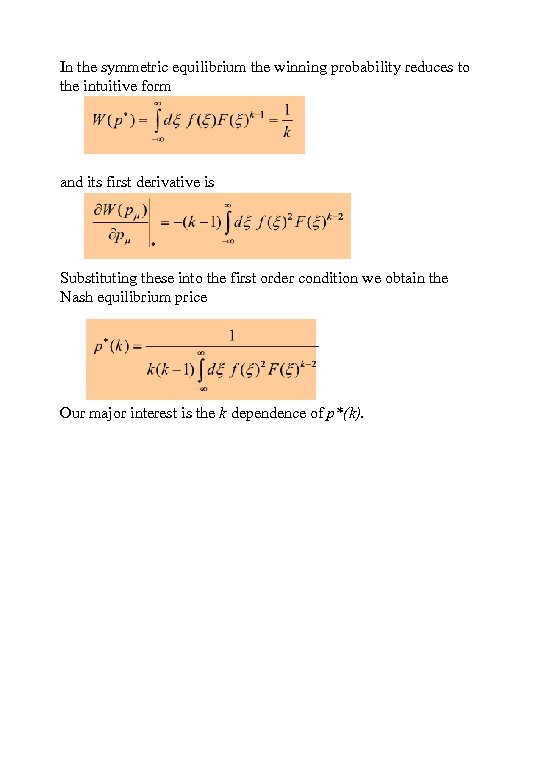In the symmetric equilibrium the winning probability reduces to the intuitive form and its first derivative is Substituting these into the first order condition we obtain the Nash equilibrium price Our major interest is the k dependence of p*(k).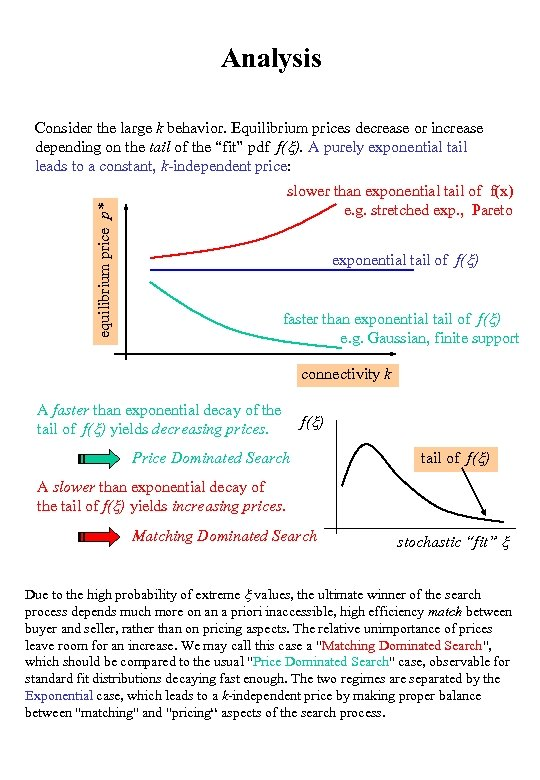Analysis equilibrium price p* Consider the large k behavior. Equilibrium prices decrease or increase depending on the tail of the “fit” pdf f(x). A purely exponential tail leads to a constant, k-independent price: slower than exponential tail of f(x) e. g. stretched exp. , Pareto exponential tail of f(x) faster than exponential tail of f(x) e. g. Gaussian, finite support connectivity k A faster than exponential decay of the f(x) tail of f(x) yields decreasing prices. Price Dominated Search tail of f(x) A slower than exponential decay of the tail of f(x) yields increasing prices. Matching Dominated Search stochastic “fit” x Due to the high probability of extreme x values, the ultimate winner of the search process depends much more on an a priori inaccessible, high efficiency match between buyer and seller, rather than on pricing aspects. The relative unimportance of prices leave room for an increase. We may call this case a "Matching Dominated Search", which should be compared to the usual "Price Dominated Search" case, observable for standard fit distributions decaying fast enough. The two regimes are separated by the Exponential case, which leads to a k-independent price by making proper balance between "matching" and "pricing“ aspects of the search process.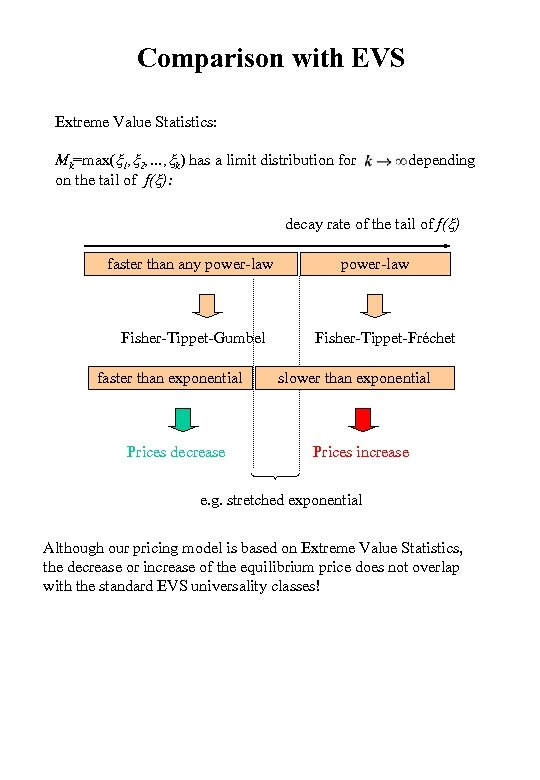Comparison with EVS Extreme Value Statistics: Mk=max(x 1, x 2, …, xk) has a limit distribution for depending on the tail of f(x): decay rate of the tail of f(x) faster than any power-law Fisher-Tippet-Gumbel faster than exponential Prices decrease power-law Fisher-Tippet-Fréchet slower than exponential Prices increase e. g. stretched exponential Although our pricing model is based on Extreme Value Statistics, the decrease or increase of the equilibrium price does not overlap with the standard EVS universality classes!